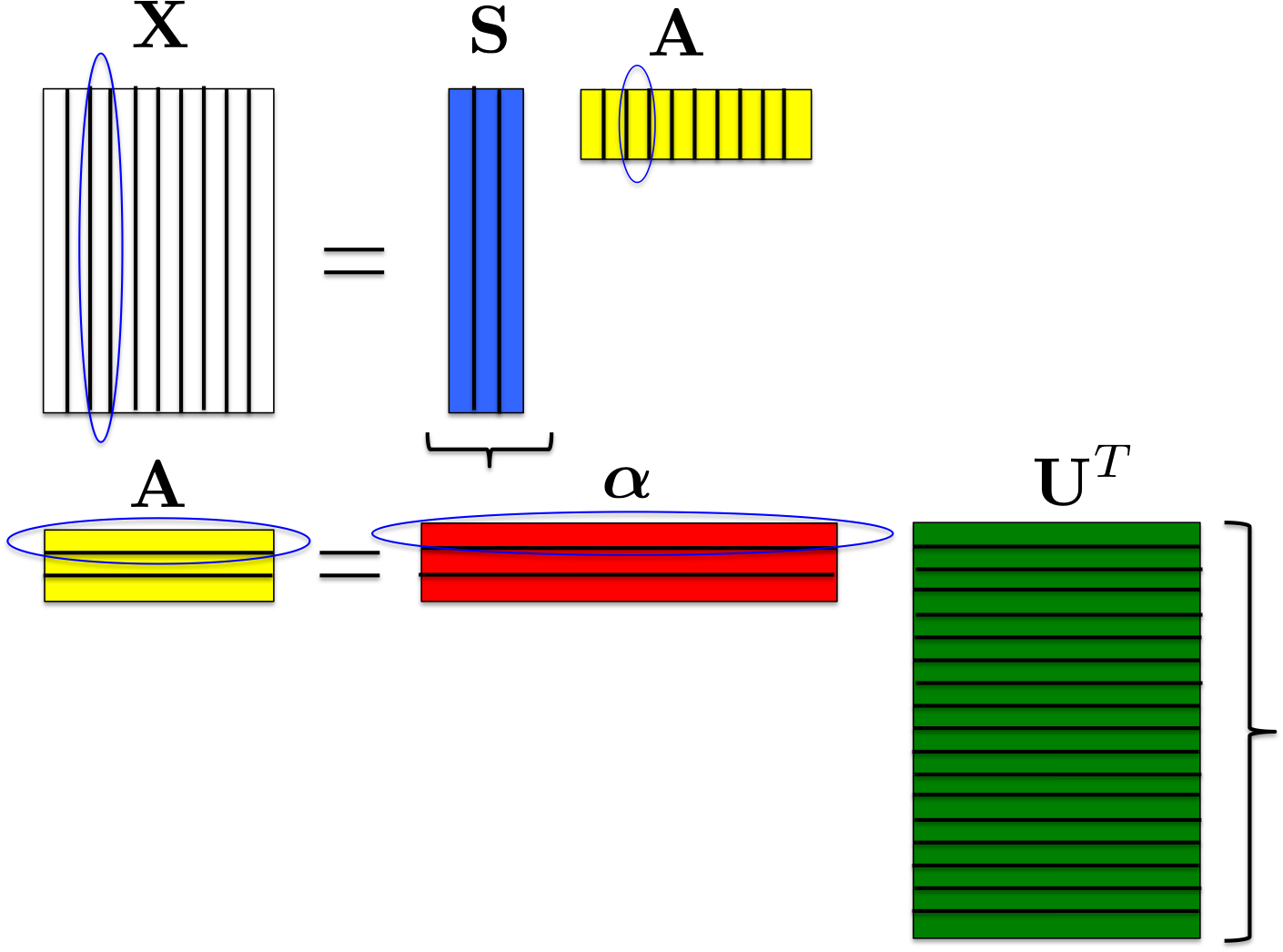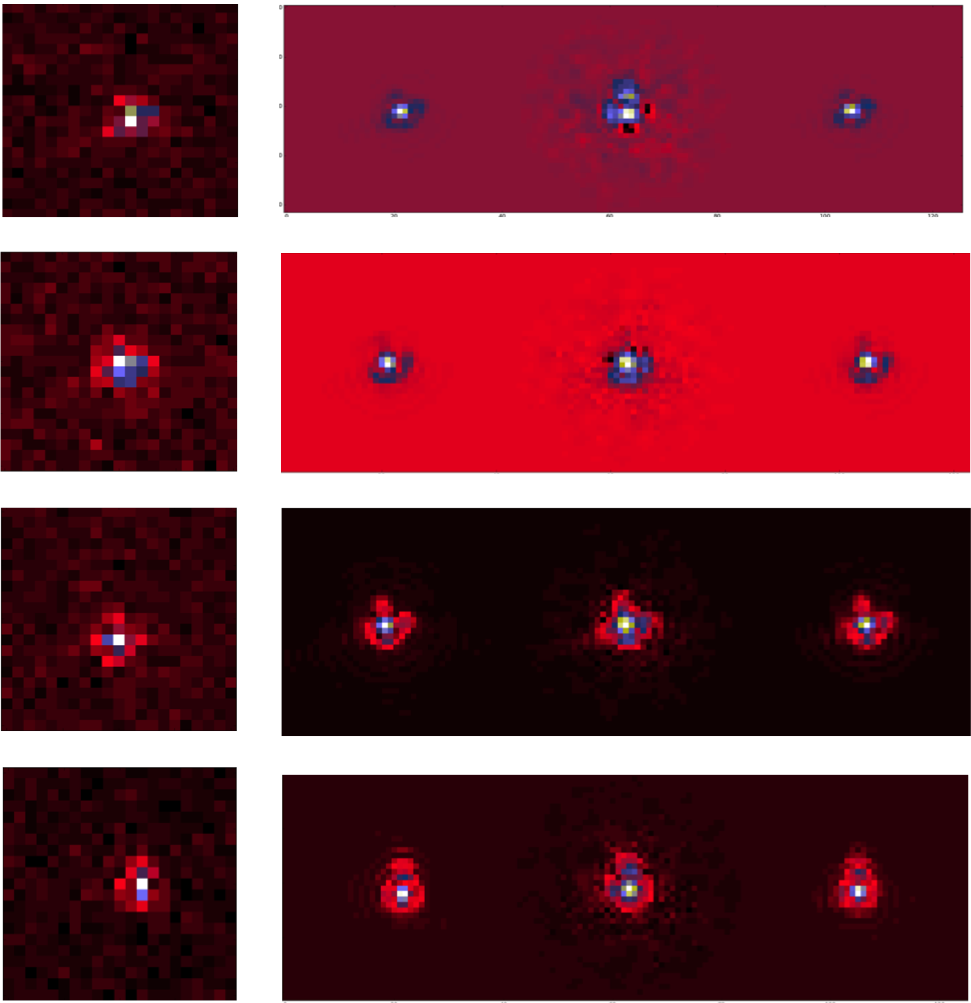RCA

 Authors: F. Ngolè-Mboula Language: Python Download: GitHub Description: RCA: sparsity-based dimension reduction and super-resolution algorithm. Notes: Packaged version available here: rca.tar.gz

• RCA: sparsity-based dimension reduction and super-resolution algorithm.

Python source code available here: rca.tar.gz

The method

RCA (Resolved Components Analysis, ) aims at characterizing globally a space-variant PSFs field. Given a set of aliased and noisy images of unresolved objects (stars images from a telescope for instance), RCA estimates well-resolved and noise-free PSFs at the observations positions, in particular, exploiting the spatial correlation of the PSFs across the imaging system field of view (FOV). Let consider a set of p images of unresolved objects $\mathbf{y}_1,\dots,\mathbf{y}_p$ in a given instrument FOV and $\mathbf{x}_1,\dots,\mathbf{x}_p$ the corresponding ideal PSFs; these images are treated as column vectors and we note $\mathbf{Y} = [\mathbf{y}_1,\dots,\mathbf{y}_p]$ and $\mathbf{X} = [\mathbf{x}_1,\dots,\mathbf{x}_p]$. RCA minimizes $\frac{1}{2}{\|\mathbf{Y}-\mathcal{F}(\mathbf{X})\|_F^2}$, where $\mathcal{F}$ accounts for the observed stars downsampling; this is done subject to several constraints over $\mathbf{X}$:

• Positivity constraint: each PSF $\mathbf{x}_j$ should be positive;
• Low rank constraint: each estimated PSF is forced to be a linear combination of a few number esimated of "eigen" PSFs; $\mathbf{x}_j = \sum_{i=1}^r a_{ij} \mathbf{s}_i$, with $r \ll p$ or equivalently, $\mathbf{X} = \mathbf{S}\mathbf{A}$, $\mathbf{S}$ and $\mathbf{A}$ being low rank matrices;
• Piece-wise smoothness constraint: we can assume that the vectors $\mathbf{x}_j$ are structured; promoting the sparsity of the "eigen" PSFs $\mathbf{s}_j$ in an appropriate dictionary $\boldsymbol{\Phi}$ allows to capture the spatial correlations within the PSFs themselves;
• Proximity constraints: the more two PSFs are close in the FOV, the more they should be similar; the p values relative to the $i^{th}$ line of $\mathbf{A}$ correspond to the contribution of the $i^{th}$ "eigen" PSF across the field of view; therefore the PSFs field regularity is enforced by constraining $\mathbf{A}$'s lines; specifically, each line is calculated as a sparse linear combination of spatial frequencies atoms: $\mathbf{A} = \boldsymbol{\alpha}\mathbf{U}^T$ (see Fig.1).Figure 1: Data matrix factorization. \mathbf{U} columns correspond to different spatial frequencies of the PSFs field.

Hence RCA solves the following problem:

The operator $\mathcal{F}$ is set according to the resolution enhancement factor specified. Besides, this operator also accounts for the observed images subpixel offsets which are automatically estimated. $\boldsymbol{\Phi}$ is a user-chosen redundant dictionary. The frequency dictionary $\mathbf{U}$ is automatically built based on the observations $\mathbf{y}_i$ locations in the imaging instrument FOV. The sparsity parameters $(\mathbf{w}_i)_i$ and $(\eta_l)_l$ as well are automatically selected. The number of "eigen" PSFs, r, has to be specified. It might be automatically reduced depending of the signal-to-noise ratio, the observations resolution and the sample size. A principal components analysis should provide the user a good first guess for r.

Numerical examples

RCA was tested on set on 500 Euclid telescope simulated PSFs. The simulated observables were downsampled to Euclid telescope resolution and corrupted with a white gaussian noise. Four examples of recovered PSFs are given in Fig.2. In this example, 10 "eigen" PSFs were required. We compare the result to the PSFs obtained from the software PSFEx, using the same observations.Figure 2: Euclid PSFs restoration. From the left to the right: observed PSFs, original PSFs, PSFEx restored PSFs, RCA restored PSFs.

As it can be seen from these reconstructions, RCA handles the undersampling and the PSFs spatial variability, with both noise-robustness and a high visual quality.

Article

•  F. M. Ngolè Mboula, J.-L. Starck, K. Okomura, J. Amiaux, P. Hudelot. Constraint matrix factorization for space variant PSFs field restoration, Inverse Problems. Available here.

SPRITE

 Authors: F. Ngolè-Mboula Language: C++ Download: sprite_v1.tgz Description: SPRITE: sparsity-based super-resolution algorithm Notes:

SPRITE: sparsity-based super-resolution algorithm

The method

SPRITE (Sparse Recovery of InstrumenTal rEsponse, 1) aims at computing a well-resolved compact source image from several undersampled and noisy observations. SPRITE solves the succession of problems of the form

with $l=1..N$.
The vectors $\mathbf{y}_k$ are the low resolution (LR) observations. The scalars $f_k$ account for possible luminosity differences between the LR images and $\sigma_k^2$ is the noise variance in the LR image $k^{th}$. The matrices $\mathbf{H}_k$ account for the shifts between the observations and the matrix D is the downsampling operator. The vector $\mathbf{x}^{(0)}$ is a rough estimate of the well-resolved image. The final image is given by $\mathbf{x}=\mathbf{x}^{(0)}+\Delta_N$, where $\Delta_N$ is the minimizer of the $N^{th}$ problem solved.

$\Phi$ is a user-chosen redundant dictionary. The method relies on the prior that a suitable solution $\Delta$ should have a sparse decomposition into the dictionary $\Phi$. Finally the inequality constraint (which is element-wise) insures that the final well-resolved image has positive pixels values.

It's important to note that the only parameters to be provided by the user are $Phi$ and $kappa$. The other parameters are automatically calculated.

The source code can be downloaded here: SPRITE package. The data and IDL codes used to perform the benchmark tests presented in 1 are available here: super-resolution benchmark.

Please cite the reference below if you use these codes in a publication. This is a preliminary version of the SPRITE package, which may be imperfect. Please feel free to contact us if you have any problem.

Article

•  F. M. Ngolè Mboula, J.-L. Starck, S. Ronayette, K. Okomura, J. Amiaux. Super-resolution method using sparse regularization for point spread function recovery, Astronomy and Astrophysics, 2014. Available here.

PSF field learning based on Optimal Transport Distances

 Authors: F. Ngolè Mboula, J-L. Starck Journal: arXiv Year: 2017 Download: ADS | arXiv

Abstract

Context: in astronomy, observing large fractions of the sky within a reasonable amount of time implies using large field-of-view (fov) optical instruments that typically have a spatially varying Point Spread Function (PSF). Depending on the scientific goals, galaxies images need to be corrected for the PSF whereas no direct measurement of the PSF is available. Aims: given a set of PSFs observed at random locations, we want to estimate the PSFs at galaxies locations for shapes measurements correction. Contributions: we propose an interpolation framework based on Sliced Optimal Transport. A non-linear dimension reduction is first performed based on local pairwise approximated Wasserstein distances. A low dimensional representation of the unknown PSFs is then estimated, which in turn is used to derive representations of those PSFs in the Wasserstein metric. Finally, the interpolated PSFs are calculated as approximated Wasserstein barycenters. Results: the proposed method was tested on simulated monochromatic PSFs of the Euclid space mission telescope (to be launched in 2020). It achieves a remarkable accuracy in terms of pixels values and shape compared to standard methods such as Inverse Distance Weighting or Radial Basis Function based interpolation methods.

Constraint matrix factorization for space variant PSFs field restoration

 Authors: F. Ngolè Mboula, J-L. Starck, K. Okumura, J. Amiaux, P. Hudelot Journal: IOP Inverse Problems Year: 2016 Download: ADS | arXiv

Abstract

Context: in large-scale spatial surveys, the Point Spread Function (PSF) varies across the instrument field of view (FOV). Local measurements of the PSFs are given by the isolated stars images. Yet, these estimates may not be directly usable for post-processings because of the observational noise and potentially the aliasing. Aims: given a set of aliased and noisy stars images from a telescope, we want to estimate well-resolved and noise-free PSFs at the observed stars positions, in particular, exploiting the spatial correlation of the PSFs across the FOV. Contributions: we introduce RCA (Resolved Components Analysis) which is a noise-robust dimension reduction and super-resolution method based on matrix factorization. We propose an original way of using the PSFs spatial correlation in the restoration process through sparsity. The introduced formalism can be applied to correlated data sets with respect to any euclidean parametric space. Results: we tested our method on simulated monochromatic PSFs of Euclid telescope (launch planned for 2020). The proposed method outperforms existing PSFs restoration and dimension reduction methods. We show that a coupled sparsity constraint on individual PSFs and their spatial distribution yields a significant improvement on both the restored PSFs shapes and the PSFs subspace identification, in presence of aliasing. Perspectives: RCA can be naturally extended to account for the wavelength dependency of the PSFs.

Super-resolution method using sparse regularization for point-spread function recovery

 Authors: F. Ngolè Mboula, J-L. Starck, S. Ronayette, K. Okumura, J. Amiaux Journal: A&A Year: 2015 Download: ADS | arXiv

Abstract

In large-scale spatial surveys, such as the forthcoming ESA Euclid mission, images may be undersampled due to the optical sensors sizes. Therefore, one may consider using a super-resolution (SR) method to recover aliased frequencies, prior to further analysis. This is particularly relevant for point-source images, which provide direct measurements of the instrument point-spread function (PSF). We introduce SPRITE, SParse Recovery of InsTrumental rEsponse, which is an SR algorithm using a sparse analysis prior. We show that such a prior provides significant improvements over existing methods, especially on low SNR PSFs.